Relation-Shape Convolutional Neural Network for Point Cloud Analysis

CVPR 2019Oral & Best paper finalist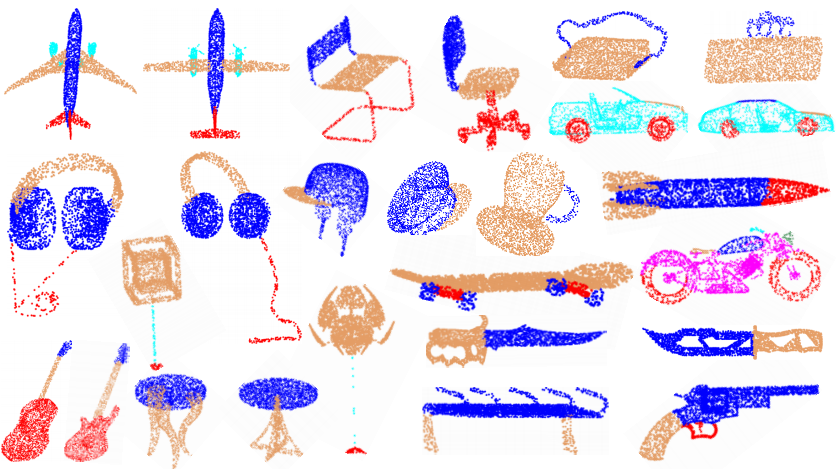Segmentation examples on ShapeNet part benchmark. Although the part shapes implied in irregular points are extremely diverse and they may be very confusing to recognize, our RS-CNN can also segment them out with decent accuracy.

Abstract

Point cloud analysis is very challenging, as the shape implied in irregular points is difficult to capture. In this paper, we propose RS-CNN, namely, Relation-Shape Convolutional Neural Network, which extends regular grid CNN to irregular configuration for point cloud analysis. The key to RS-CNN is learning from relation, i.e., the geometric topology constraint among points. Specifically, the convolutional weight for local point set is forced to learn a high-level relation expression from predefined geometric priors, between a sampled point from this point set and the others. In this way, an inductive local representation with explicit reasoning about the spatial layout of points can be obtained, which leads to much shape awareness and robustness. With this convolution as a basic operator, RS-CNN, a hierarchical architecture can be developed to achieve contextual shape-aware learning for point cloud analysis. Extensive experiments on challenging benchmarks across three tasks verify RS-CNN achieves the state of the arts.

Motivation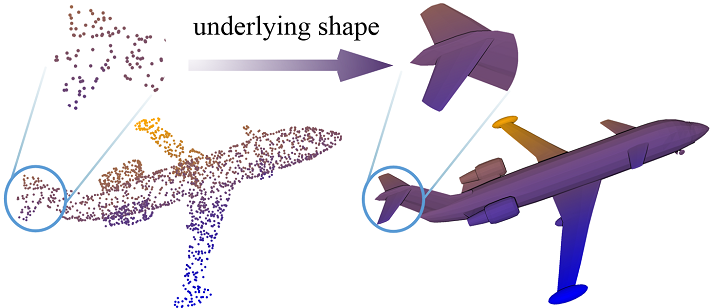Left part: 3D Point cloud. Right part: Underlying shape formed by this point cloud.

• The geometric relation among points is an explicit expression about the spatial layout of points, further discriminatively reflecting the underlying shape.

• CNN has demonstrated its powerful visual abstraction capability for 2D images that are in the format of a regular grid.

• Can we extend 2D grid CNN to 3D irregular configuration for point cloud analysis, by learning expressive geometric relation encoding for discriminative shape awareness?

RS-Conv: Relation-Shape Convolution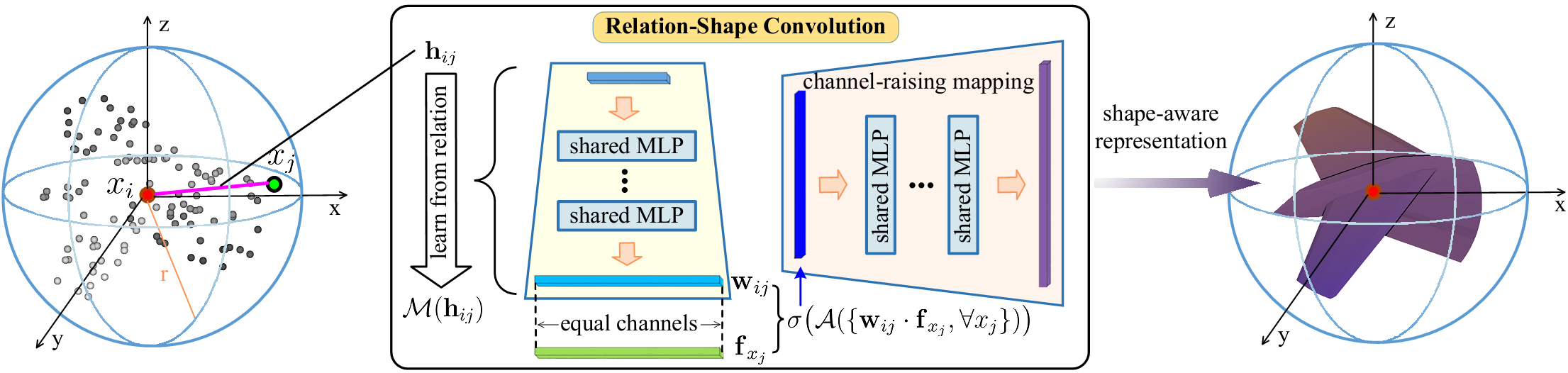Overview of our relation-shape convolution (RS-Conv).

In this paper, we develop a hierarchical CNN-like architecture, i.e. RS-CNN. RS-CNN is equipped with a novel learn-from-relation convolution operator called relation-shape convolution (RS-Conv). As illustrated in the figure, the key to RS-CNN is learning from relation.

To be specific:

• The convolutional weight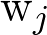for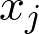is converted to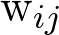, which learns a high-level mapping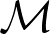, i.e.,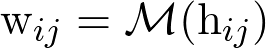, on predefined geometric relation vector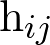.

• In this way, the inductive convolutional representation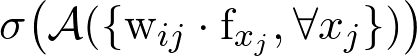can expressively reason the spatial layout of points, resulting in discriminative shape awareness.

• As in image CNN, further channel-raising mapping is conducted for a more powerful shape-aware representation.

Revisiting 2D Grid Convolution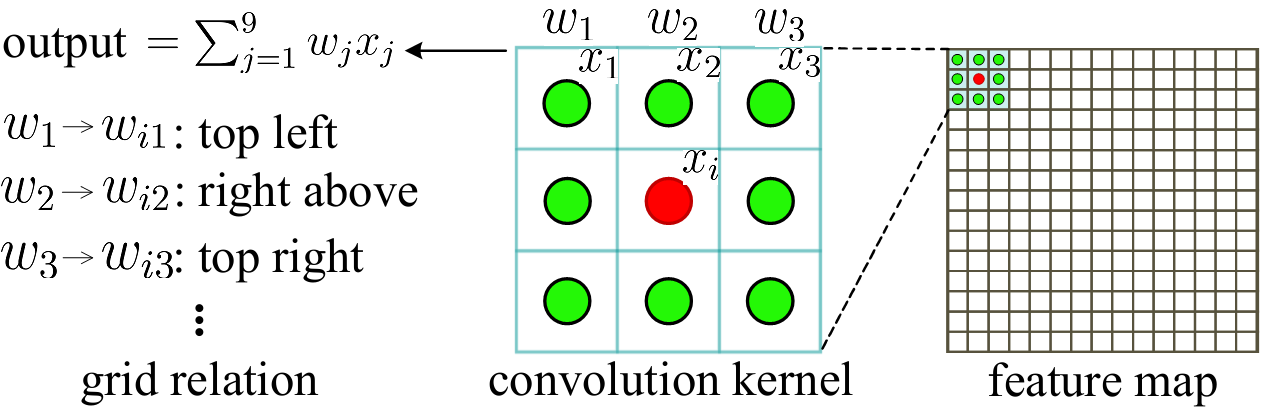Illustration of 2D grid convolution with a kernel of 3 x 3.

• The convolutional weight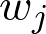foralways implies a fixed positional relation between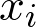and its neighborin the regular grid. That is,is actually constrained to encode one kind of regular grid relation in the learning process.

• Therefore, our RS-Conv with relation learning is more general and can be applied to model 2D grid spatial relationship.

Experiment

Shape Classification on ModelNet40 Benchmark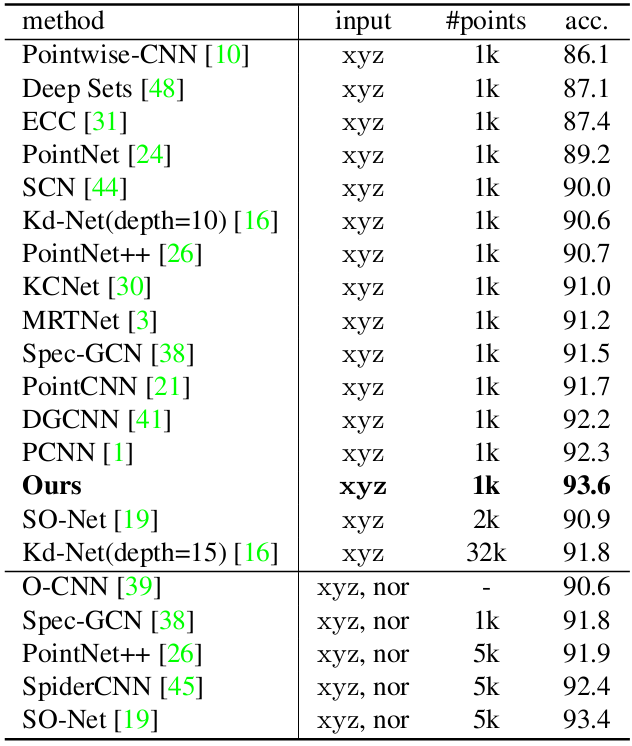Shape classification results (%) (nor: normal).

• Our RS-CNN outperforms the state-of-the-art point cloud-based methods with only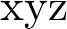as the input features.

Normal Estimation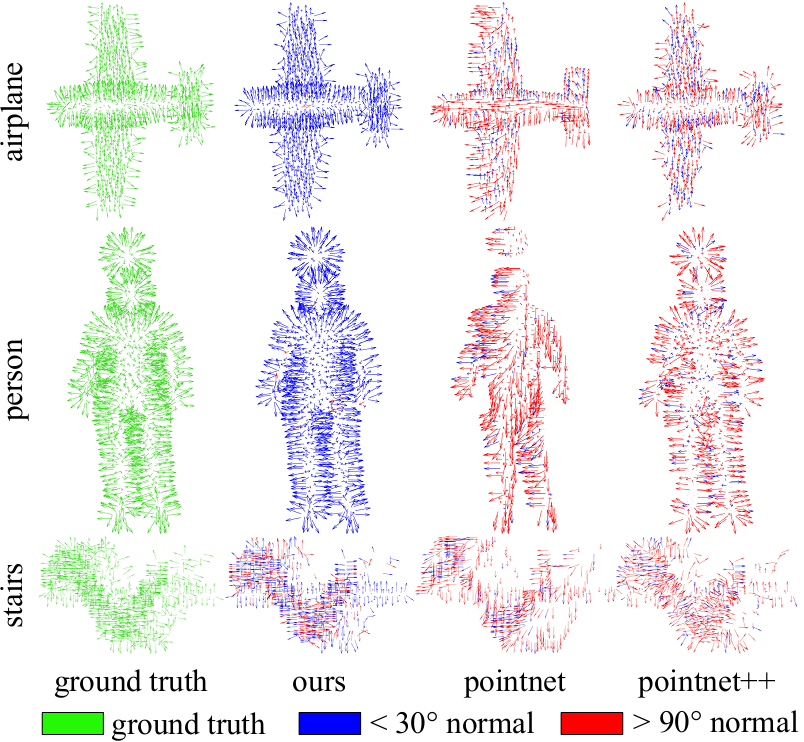Normal estimation examples. For clearness, we only show predictions with angle less than 30 degree in blue, and angle greater than 90 degree in red between the ground truth normals.

Geometric Relation Definition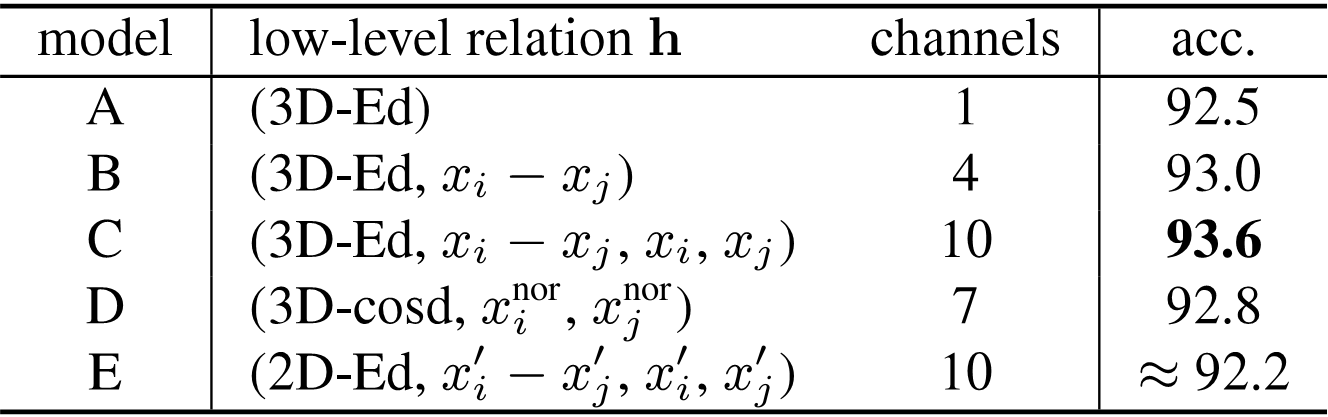The results (%) of five intuitive low-level relation. Model A applies only 3D Euclidean distance; Model B adds the coordinates difference to model A; Model C adds the coordinates of two points to model B; Model D utilizes the normals of two points and their cosine distance; Model E projects 3D points onto a 2D plane of XY, XZ and YZ.

• The low-level relation vector can be defined flexibly.

• Using only 3D Euclidean distance as relation can result in an accuracy of 92.5%.

• Even learning from the relation in 2D projections of points, a decent performance of 92.2% can also be achieved.

Robustness to sampling density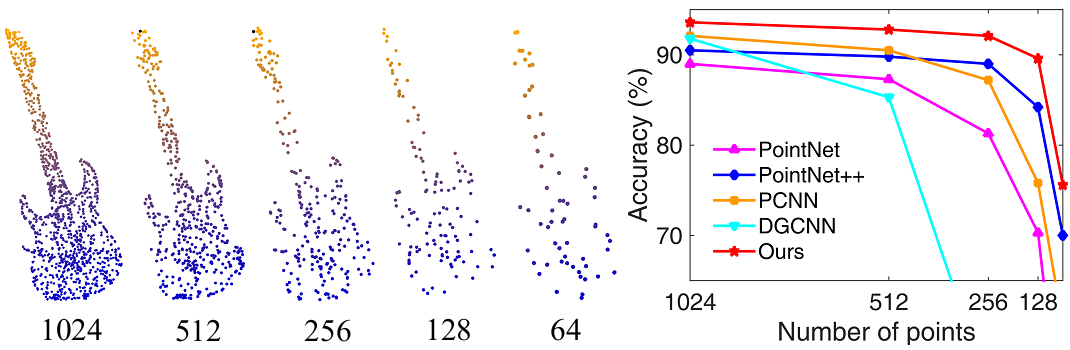Left part: Point cloud with random point dropout. Right part: Test results of using sparser points as the input to a model trained with 1024 points.

Robustness to point permutation and rigid transformation (%)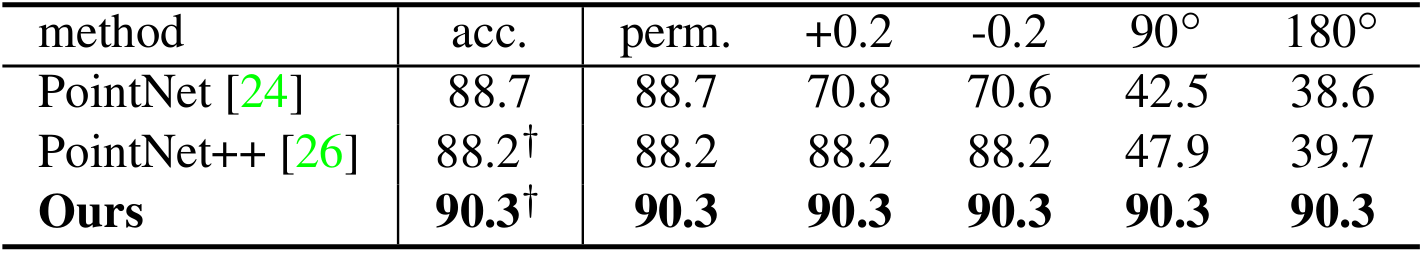All the models are trained without related data augmentations, e.g., translation or rotation, to avoid confusion. During testing, we perform random permutation (perm.) of points, add a small translation of 0.2 and rotate the input point cloud by 90 degree and 180 degree.

Visualization and Complexity

Visualization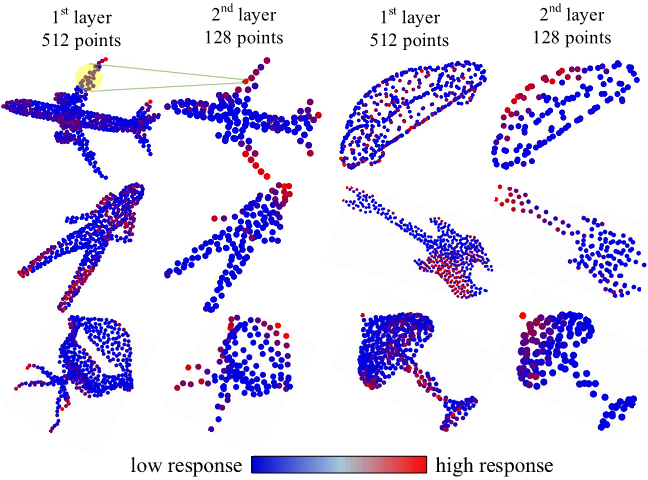Visualization of the shape features learned by the first two layers of RS-CNN.

• The features learned by the first layer mostly respond to edges, corners and arcs, while the ones in the second layer capture more semantical shape parts like airfoils and heads.

• As image CNN, our RS-CNN learns 3D shape semantics from point cloud in a local-to-global manner.

Complexity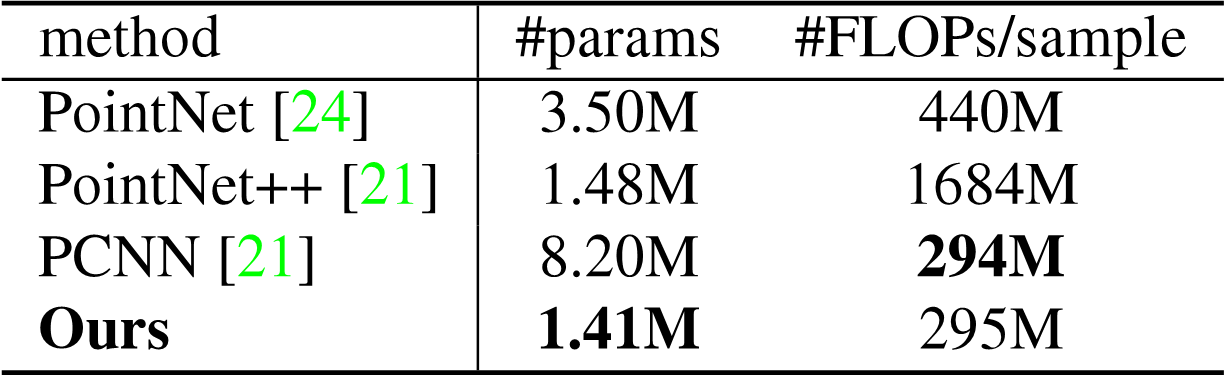Complexity of RS-CNN in point cloud classification.

Publication

Yongcheng Liu, Bin Fan, Shiming Xiang and Chunhong Pan, “Relation-Shape Convolutional Neural Network for Point Cloud Analysis”, in IEEE Conference on Computer Vision and Pattern Recognition (CVPR), 2019. [arXiv] [CVF]

@inproceedings{liu2019rscnn,
author = {Yongcheng Liu and
Bin Fan and
Shiming Xiang and
Chunhong Pan},
title = {Relation-Shape Convolutional Neural Network for Point Cloud Analysis},
booktitle = {IEEE Conference on Computer Vision and Pattern Recognition (CVPR)},
pages = {8895--8904},
year = {2019}
}